#Function Repository Resource:

# CloudPutByHash

CloudPut to a location determined by the hash of an expression

Contributed by: Richard Hennigan (Wolfram Research)
 ResourceFunction["CloudPutByHash"][expr] CloudPut expr to a CloudObject determined by the hash of expr. ResourceFunction["CloudPutByHash"][expr,root] uses root as the base directory in the cloud.

## Examples

### Basic Examples

CloudPut an expression:

 In:=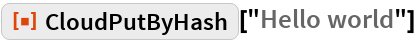Out=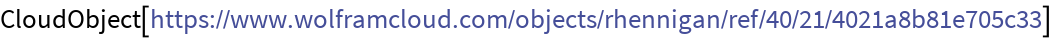The same expression will produce the same cloud object:

 In:=Out=In:=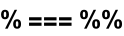Out=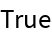### Scope

Specify a different root directory:

 In:=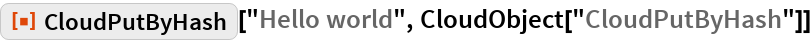Out=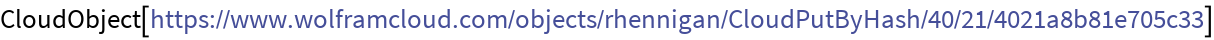### Generalizations and Extensions

The hash can be different for unevaluated expressions:

 In:=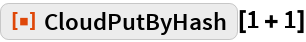Out=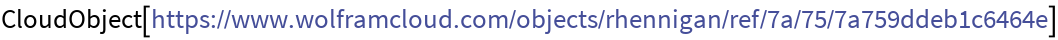In:=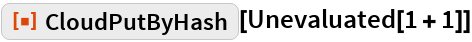Out=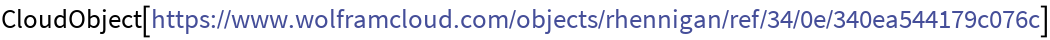Expressions are compressed:

 In:=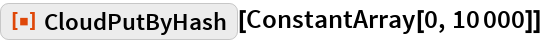Out=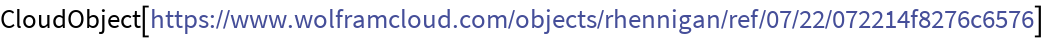In:=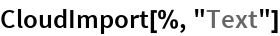Out=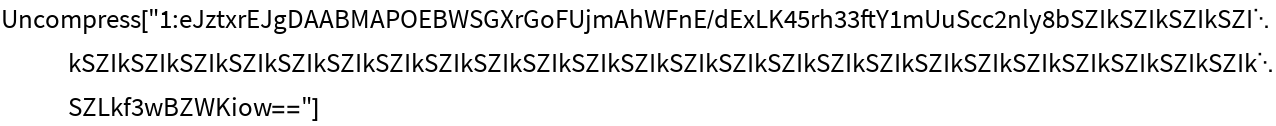In:=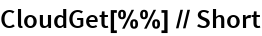Out=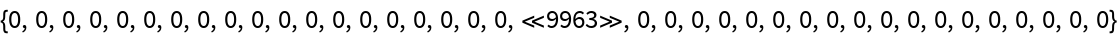## Requirements

Wolfram Language 11.3 (March 2018) or above